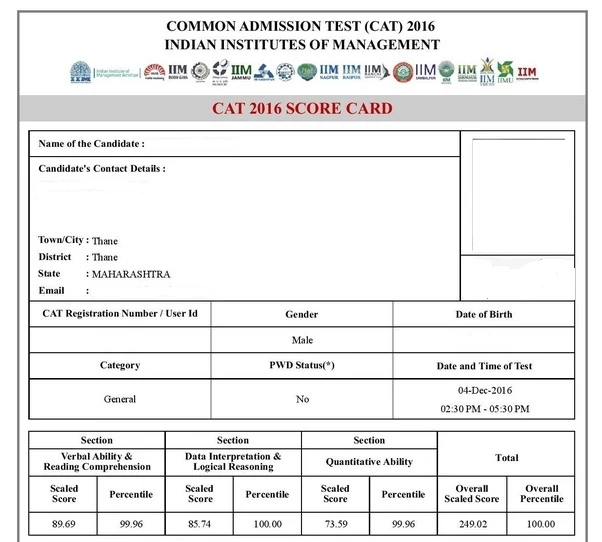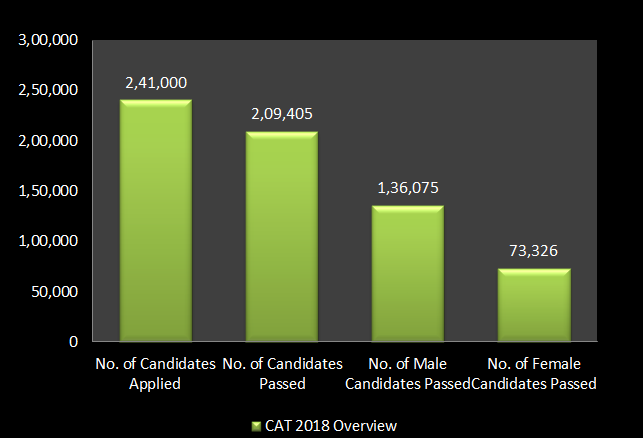### CAT 2022 Result:

The result for CAT 2022 will be declared by the authorities online. The students can check their result by logging in to the official website of CAT 2022. The result will be available in the form of a scorecard. On the basis of the results, the candidates can apply to the colleges according to the CAT 2022 cutoff set by the institutions. They must keep a check on the deadline for applying to the colleges. The scores will be valid for one year

#### How To Check CAT 2022 Result Online?

• Visit the official website of CAT 2022

After the results, the candidate will be called for the further rounds of admission and CAT 2022 Counselling process. The candidate can check the top colleges accepting the CAT score and apply accordingly.

The scoring of the CAT is based on 3 patterns:

• Raw CAT mark
• Scaled or normalized mark
• CAT percentile

#### 1. Raw CAT Mark

• CAT  consists of a total of 66 questions:
• Verbal Ability & Reading Comprehension (VARC) - 24 question
• Data Interpretation & Logical Reasoning (DILR) - 20 question
• Quantitative Ability (QA) - 22 question

The CAT exam consists of both MCQs and Non-MCQs type of question. Each correct answer is awarded by +3 marks and each incorrect answer results in the deduction of -1 mark. So the total mark for the 66 questions is 198. The raw CAT mark is referred to as the total mark score from a total of 198 marks.

#### 2. Scaled or normalized mark

The raw mark is then converted into scaled or normalized marks. The CAT normalization process ensures fairness and equality in performance comparison across sessions. This process is also used by GATE. Various factors are taken into consideration while converting raw marks into scaled marks like, no. of. candidates appearing in the exam in the given year, the difficulty level of the exam, the slot number the candidate is appearing, etc. This scaled mark is then converted into a percentile.

The formula for obtaining the scaled mark of a candidate is:

S =  (Ar-G)*(A1-G1) / (Am1-G1) + G

where,

S= Scaled Mark

R = Actual or raw marks obtained by the candidate

Ar = average mark obtained of the top 0.1% candidates in both slots

G = Sum of the mean and standard deviation of marks of all candidates in both slots

Am1 = Average mark of top 0.1% candidates in ith slot

G1 = Sum of mean and deviation mark of all the candidates in ith slot

Example:

Total Slots = 2

Let,

Total test takers = 2,00,000

Candidates in each slot = 1,00,000 (O.1% = 100)

R = 170 out 0f 300

Ar = (195 + 190)/2 = 192, (where 195 and 190 are average mark of top 100 candidates in slot 1 and 2 respectively)

G = 112

Am1 = 190 (let consider 1st slot)

G1 = 110

So Scaled Mark S = (192-112)*(170-110) / (190-110) + 112 = 172

3. CAT 2022 Percentile

The CAT  percentile is referred to as the total percentage of candidates who scored less than or equal to a particular candidate. This means if a candidate scores 99 percentile, then his/her' performance was better than 99% of the total candidate that appeared CAT that particular year. To clear this confusion, the Cat percentile is not referred to the actual mark obtained by the candidate but it refers to the candidate's performance in comparison to the rest of the CAT aspirants.

The scaled score and corresponding percentile depends on the level of difficulty of the exam and the number of test-takers

#### Details Mentioned in the CAT 2022 Scorecard:

• CAT 2022 registration number/user Id
• Candidate's name, category, and gender
• Date of birth
• Date and time of examination
• Candidate contact details and email id
• CAT 2022 scaled score (overall)
• CAT 20202score (section-wise)
• CAT 2022 percentile score (section-wise)
• CAT 2022 percentile score (overall)

#### Sample of a CAT Score Card:#### CAT 2018 OverviewRead Also: CAT Score Vs CAT Percentile - Get Clear of Your Confusion Forever